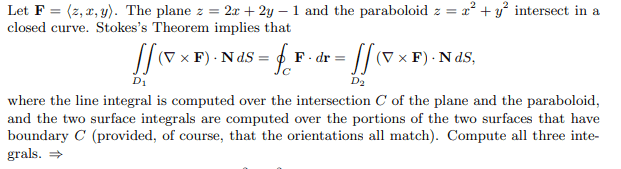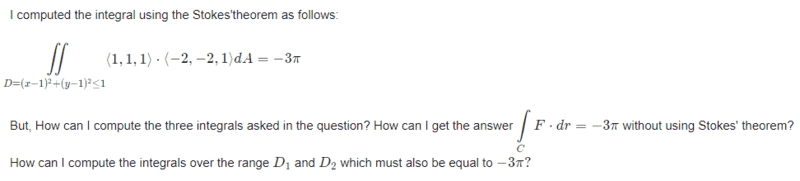# Line integrals and Stokes' theorem

• WMDhamnekar
In summary, the conversation is about calculating integrals using parametrization and verifying the use of Stokes' theorem in a specific case. The expert suggests forgetting about the theorem and just calculating the integrals, and also provides a suggestion for correcting an incorrect parametrization. The conversation also includes a note on using correct formatting in LaTeX.f

#### WMDhamnekar

MHB
Homework Statement
https://mathhelpforum.com/attachments/1667128375169-png.45062/
Relevant Equations
##F=\langle z,x,y \rangle, z = 2x + 2y -1## and ##z = x^2 + y^2 ##Please type out your attempts rather than post an image. Posting an image makes it more difficult to read and makes it impossible to quote particular parts of it.

To answer your question: You would just compute the integrals as usual.

••WMDhamnekar and topsquark
You haven't used Stokes' theorem. You just calculated the first integral. (I assume. I didn't check your work.) Stokes' theorem tells you that if you were to calculate the other integrals, you will get the same result. So in this problem, you're really just verifying that the theorem works in this particular case, not actually using it.

So forget about using the theorem and just calculate the other integrals.

•topsquark and haider
Just curious, which book is this problem from?

You haven't used Stokes' theorem. You just calculated the first integral. (I assume. I didn't check your work.) Stokes' theorem tells you that if you were to calculate the other integrals, you will get the same result. So in this problem, you're really just verifying that the theorem works in this particular case, not actually using it.

So forget about using the theorem and just calculate the other integrals.
I computed line integral as follows:
Curve ##D = (x-1)^2 + (y-1)^2 = 1## Now I parametrize it ##x = \cos(t) , y= \sin(t) , z = 2\cos(t) + 2\sin(t) ##

##F (x,y,z) = zdx + xdy +y dz, r(t) = (\cos(t))i + (\sin(t))j +(\cos(t) +\sin(t))k , x'(t) = - \sin(t), y'(t)= \cos(t), z'(t)= -2\sin(t) +2\cos(t) ##
##\begin{align*}\displaystyle\int_ D F\cdot dr &= \displaystyle\int_0^{2\pi} (2\cos(t)+ 2\sin(t))(-\sin(t)) +(\cos(t))(\cos(t)+(\sin(t))(-2\sin(t) + 2\cos(t))dt\\
&= \displaystyle\int_0^{2\pi} \cos^2(t)-4\sin^2(t)-4\sin(t)\cos(t) dt\\
&= -3\pi \end{align*}##

Last edited:
Your parametrization does not describe the correct curve.

•WMDhamnekar
Your parametrization does not describe the correct curve.
If my parametrization of the curve is wrong, what is your suggestion for correct parametrization of the curve?

If my parametrization of the curve is wrong, what is your suggestion for correct parametrization of the curve?
I believe it would be more instructive if you first verified that it is indeed wrong and then think about what you could do to correct it. What do you get if you insert the parametrization in, for example, the equation for the paraboloid ##z = x^2 + y^2## or the plane ##z = 2x + 2y - 1##?

Edit: Or indeed into ##(x-1)^2 + (y-1)^2 = 1## ...

Edit 2: On a TeXnical note: Please use \cos and \sin for the trigonometric functions in LaTeX. Just writing sin and cos makes LaTeX typest it as if it was a product of ##s##, ##i##, and ##n## and ##c##, ##o##, and ##s##, respectively. Note the difference:
$$sin x \leftrightarrow \sin x \qquad cos x \leftrightarrow \cos x$$